# Applying Research

## Long Short-Term Memory

### Introduction

This page explains how to you can use the Research Environment to develop and test a Long Short Term Memory hypothesis, then put the hypothesis in production.

Recurrent neural networks (RNN) are a powerful tool in deep learning. These models quite accurately mimic how humans process sequencial information and learn. Unlike traditional feedforward neural networks, RNNs have memory. That is, information fed into them persists and the network is able to draw on this to make inferences.

Long Short-term Memory (LSTM) is a type of RNN. Instead of one layer, LSTM cells generally have four, three of which are part of "gates" -- ways to optionally let information through. The three gates are commonly referred to as the forget, input, and output gates. The forget gate layer is where the model decides what information to keep from prior states. At the input gate layer, the model decides which values to update. Finally, the output gate layer is where the final output of the cell state is decided. Essentially, LSTM separately decides what to remember and the rate at which it should update.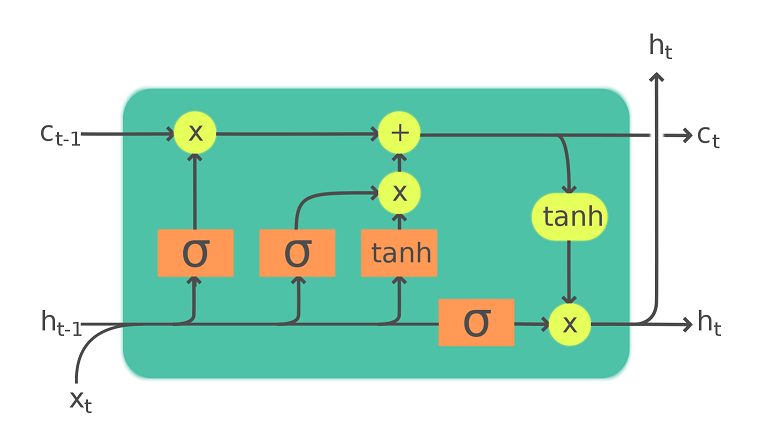An exmaple of a LSTM cell: x is the input data, c is the long-term memory, h is the current state and serve as short-term memory, $\sigma$ and $tanh$ is the non-linear activation function of the gates.
Image source: https://en.wikipedia.org/wiki/Long_short-term_memory#/media/File:LSTM_Cell.svg

### Create Hypothesis

LSTM models have produced some great results when applied to time-series prediction. One of the central challenges with conventional time-series models is that, despite trying to account for trends or other non-stationary elements, it is almost impossible to truly predict an outlier like a recession, flash crash, liquidity crisis, etc. By having a long memory, LSTM models are better able to capture these difficult trends in the data without suffering from the level of overfitting a conventional model would need in order to capture the same data.

For a very basic application, we're hypothesizing LSTM can offer an accurate prediction in future price.

### Import Libraries

We'll need to import libraries to help with data processing, validation and visualization. Import keras, sklearn, numpy and matplotlib libraries by the following:

from keras.layers import LSTM, Dense, Dropout
from keras.models import Sequential
from keras.callbacks import EarlyStopping
from sklearn.preprocessing import MinMaxScaler

import numpy as np
from matplotlib import pyplot as plt

### Get Historical Data

To begin, we retrieve historical data for researching.

1. Instantiate a QuantBook.
2. qb = QuantBook()
3. Select the desired index for research.
4. asset = "SPY"
5. Call the AddEquity method with the tickers, and their corresponding resolution.
6. qb.AddEquity(asset, Resolution.Minute)

If you do not pass a resolution argument, Resolution.Minute is used by default.

7. Call the History method with qb.Securities.Keys for all tickers, time argument(s), and resolution to request historical data for the symbol.
8. history = qb.History(qb.Securities.Keys, datetime(2019, 1, 1), datetime(2021, 12, 31), Resolution.Daily)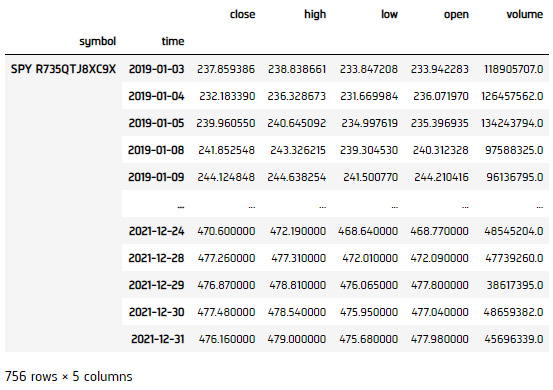### Prepare Data

We'll have to process our data as well as build the LSTM model before testing the hypothesis. We would scale our data to for better covergence.

1. Select the close column and then call the unstack method.
2. close_price = history['close'].unstack(level=0)
3. Initialize MinMaxScaler to scale the data onto [0,1].
4. scaler = MinMaxScaler(feature_range = (0, 1))
5. Transform our data.
6. df = pd.DataFrame(scaler.fit_transform(close), index=close.index)
7. Select input data
8. scaler = MinMaxScaler(feature_range = (0, 1))
9. Shift the data for 1-step backward as training output result.
10. output = df.shift(-1).iloc[:-1]
11. Split the data into training and testing sets.
12. In this example, we use the first 80% data for trianing, and the last 20% for testing.

splitter = int(input_.shape * 0.8)
X_train = input_.iloc[:splitter]
X_test = input_.iloc[splitter:]
y_train = output.iloc[:splitter]
y_test = output.iloc[splitter:]
13. Build feauture and label sets (using number of steps 60, and feature rank 1).
14. features_set = []
labels = []
for i in range(60, X_train.shape):
features_set.append(X_train.iloc[i-60:i].values.reshape(-1, 1))
labels.append(y_train.iloc[i])
features_set, labels = np.array(features_set), np.array(labels)
features_set = np.reshape(features_set, (features_set.shape, features_set.shape, 1))

### Build Model

We construct the LSTM model.

1. Build a Sequential keras model.
2. model = Sequential()
3. Create the model infrastructure.
4. # Add our first LSTM layer - 50 nodes.
model.add(LSTM(units = 50, return_sequences=True, input_shape=(features_set.shape, 1)))
# Add Dropout layer to avoid overfitting
model.add(Dense(units = 1))
5. Compile the model.
6. We use Adam as optimizer for adpative step size and MSE as loss function since it is continuous data.

model.compile(optimizer = 'adam', loss = 'mean_squared_error', metrics=['mae', 'acc'])
7. Set early stopping callback method.
8. callback = EarlyStopping(monitor='loss', patience=3, verbose=1, restore_best_weights=True)
9. Display the model structure.
10. model.summary()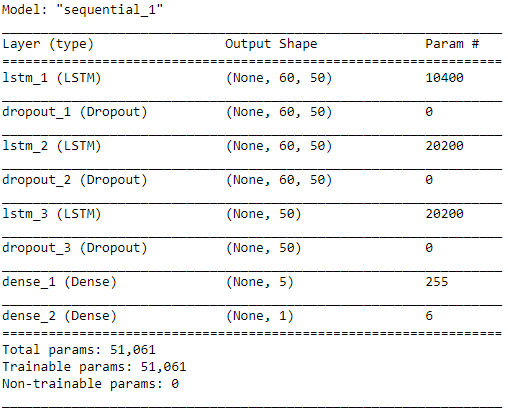11. Fit the model to our data, running 20 training epochs.
12. Note that different training session's results will not be the same since the batch is randomly selected.

model.fit(features_set, labels, epochs = 20, batch_size = 100, callbacks=[callback])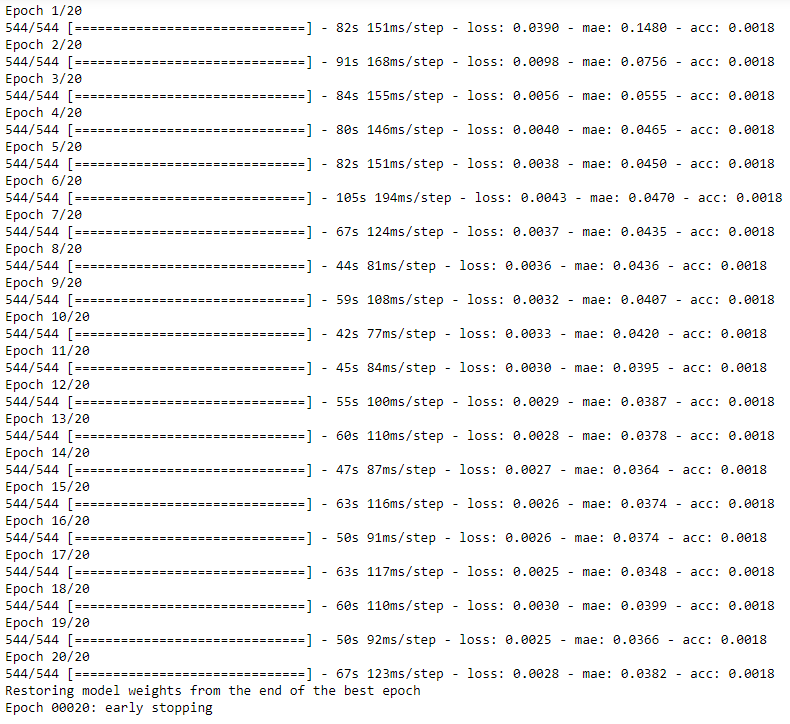### Test Hypothesis

We would test the performance of this ML model to see if it could predict 1-step forward price precisely. To do so, we would compare the predicted and actual prices.

1. Get testing set features for input.
2. test_features = []
for i in range(60, X_test.shape):
test_features.append(X_test.iloc[i-60:i].values.reshape(-1, 1))
test_features = np.array(test_features)
test_features = np.reshape(test_features, (test_features.shape, test_features.shape, 1))
3. Make predictions.
4. predictions = model.predict(test_features)
5. Transform predictions back to original data-scale.
6. predictions = scaler.inverse_transform(predictions)
actual = scaler.inverse_transform(y_test.values)
7. Plot the results.
8. plt.figure(figsize=(15, 10))
plt.plot(actual[60:], color='blue', label='Actual')
plt.plot(predictions , color='red', label='Prediction')
plt.title('Price vs Predicted Price ')
plt.legend()
plt.show()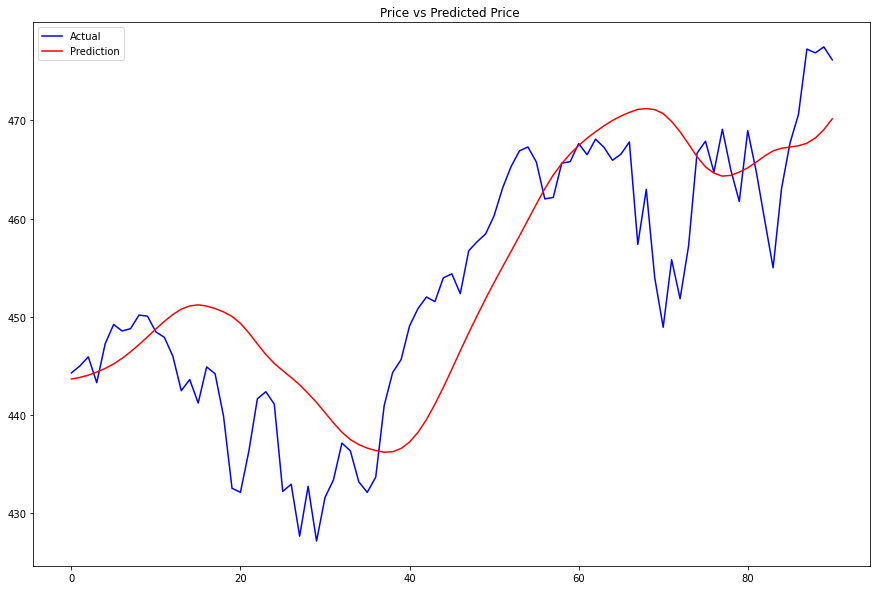### Set Up Algorithm

Once we are confident in our hypothesis, we can export this code into backtesting. One way to accomodate this model into backtest is to create a scheduled event which uses our model to predict the expected return. If we predict the price will go up, we long SPY, else, we short it.

def Initialize(self) -> None:

#1. Required: Five years of backtest history
self.SetStartDate(2016, 1, 1)

#2. Required: Alpha Streams Models:
self.SetBrokerageModel(BrokerageName.AlphaStreams)

#3. Required: Significant AUM Capacity
self.SetCash(1000000)

#4. Required: Benchmark to SPY
self.SetBenchmark("SPY")

self.asset = "SPY"

# Initialize the LSTM model
self.BuildModel()

# Set Scheduled Event Method For Our Model
self.Schedule.On(self.DateRules.EveryDay(),
self.TimeRules.BeforeMarketClose("SPY", 5),
self.EveryDayBeforeMarketClose)

# Set Scheduled Event Method For Our Model Retraining every month
self.Schedule.On(self.DateRules.MonthStart(),
self.TimeRules.At(0, 0),
self.BuildModel)

We'll also need to create a function to train and update our model from time to time.

def BuildModel(self) -> None:
qb = self

### Preparing Data
# Get historical data
history = qb.History(qb.Securities.Keys, 252*2, Resolution.Daily)

# Select the close column and then call the unstack method.
close = history['close'].unstack(level=0)

# Scale data onto [0,1]
self.scaler = MinMaxScaler(feature_range = (0, 1))

# Transform our data
df = pd.DataFrame(self.scaler.fit_transform(close), index=close.index)

# Feature engineer the data for input.
input_ = df.iloc[1:]

# Shift the data for 1-step backward as training output result.
output = df.shift(-1).iloc[:-1]

# Build feauture and label sets (using number of steps 60, and feature rank 1)
features_set = []
labels = []
for i in range(60, input_.shape):
features_set.append(input_.iloc[i-60:i].values.reshape(-1, 1))
labels.append(output.iloc[i])
features_set, labels = np.array(features_set), np.array(labels)
features_set = np.reshape(features_set, (features_set.shape, features_set.shape, 1))

### Build Model
# Build a Sequential keras model
self.model = Sequential()

# Add our first LSTM layer - 50 nodes
self.model.add(LSTM(units = 50, return_sequences=True, input_shape=(features_set.shape, 1)))
# Add Dropout layer to avoid overfitting

# Compile the model. We use Adam as optimizer for adpative step size and MSE as loss function since it is continuous data.
self.model.compile(optimizer = 'adam', loss = 'mean_squared_error', metrics=['mae', 'acc'])

# Set early stopping callback method
callback = EarlyStopping(monitor='loss', patience=3, restore_best_weights=True)

# Fit the model to our data, running 20 training epochs
self.model.fit(features_set, labels, epochs = 20, batch_size = 1000, callbacks=[callback])

Now we export our model into the scheduled event method. We will switch qb with self and replace methods with their QCAlgorithm counterparts as needed. In this example, this is not an issue because all the methods we used in research also exist in QCAlgorithm.

def EveryDayBeforeMarketClose(self) -> None:
qb = self
# Fetch history on our universe
history = qb.History(qb.Securities.Keys, 60, Resolution.Daily)
if history.empty: return

# Make all of them into a single time index.
close = history.close.unstack(level=0)

# Scale our data
df = pd.DataFrame(self.scaler.transform(close), index=close.index)

# Feature engineer the data for input
input_ = []
input_.append(df.values.reshape(-1, 1))
input_ = np.array(input_)
input_ = np.reshape(input_, (input_.shape, input_.shape, 1))

# Prediction
prediction = self.model.predict(input_)

# Revert the scaling into price
prediction = self.scaler.inverse_transform(prediction)

# ==============================

if prediction > qb.Securities[self.asset].Price:
self.SetHoldings(self.asset, 1.)
else:
self.SetHoldings(self.asset, -1.)

### Clone Example Project

You can also see our Videos. You can also get in touch with us via Discord.